# RRB ALP 2018 Practice Test Papers | Arithmetic Questions (Day-71)

Dear Aspirants, Here we have given the Important RRB ALP & Technicians Exam 2018 Practice Test Papers. Candidates those who are preparing for RRB ALP 2018 can practice these Arithmetic Questions to get more confidence to Crack RRB 2018 Examination.

[WpProQuiz 3002]

Click “Start Quiz” to attend these Questions and view Solutions

1) What approximate value will come in place of question mark in the following question?

7.999×5.202+9.099

a) 45

b) 40

c) 50

d) 55

2) Find the value of x

450%of 78 + 48% of 50 = 500 + x

a) 81

b) 72

c) 91

d) 78

3) If x/y=5 and y is not ‘0’ what % of x is 2x-y

a) 325%

b) 180%

c) 250%

d) 175%

4) A Man working 10 hours a day the work completed in 3 days. The work will be completed in how many days,if he working 12 hours a day?

a) 2 days

b) 2.5 days

c) 3 days

d) 3.5 days

5) The ratio between the speeds of two buses 8:5, if the slowest bus covers 20 km in 2 hours, then what is the speed of fastest bus?

a) 16 kmph

b) 20 kmph

c) 10 kmph

d) 5 kmph

6) 56 is what % of 1800?

a) 7 %

b) 3 %

c) 2 %

d) 5 %

7) Area of equilateral Triangle is 163. Find the perimeter of triangle

a) 24 cm

b) 36 cm

c) 30 cm

d) 36 cm

8) If the sum of two numbers is 15 and Sum of their squares is 113. Find the Multiplied value of those numbers.

a) 60

b) 54

c) 56

d) 58

9) 40%of 75+ 75% of 80 =?

a) 80

b) 120

c) 100

d) 90

10) cos3 x – 3 sin2 x.cos x= ?

a) Sin 2x

b) cos 2x

c) Sin3x

d) Cos 3x

8×5+10 = 40 + 10 = 50

450%of 78 + 48% of 50 = 500 + x

351 + 240 = 500 + x

X = 91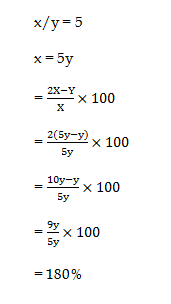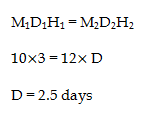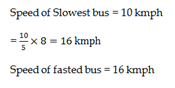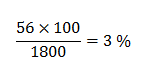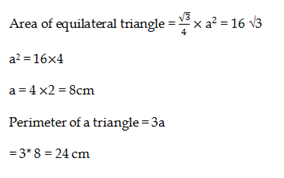Let the numbers = x, y

Sum of their numbers = (x+y) = 15

Sum of their squares = x2 + y2 = 113

(x+y)2 = x2 + y2 + 2xy

(15)2 = (113) + 2xy

225 – 113 = 2xy

2xy = 112

xy = 56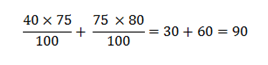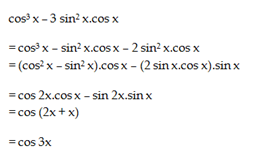RRB ALP 2018 – “All in One” Study Materials and Practice Sets